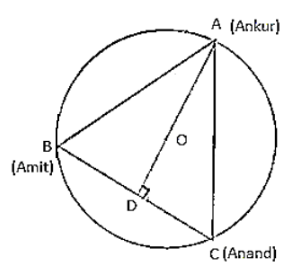# A circular park of radius 40 m is situated in a colony. Three boys Ankur,Question:

A circular park of radius 40 m is situated in a colony. Three boys Ankur, Amit and Anand are sitting at equal distance on its boundary each having a toy telephone in his hands to talk to each other. Find the length of the string of each phone.

Solution:

Given that AB = BC = CA

So, ABC is an equilateral triangle

Medians of equilateral triangle pass through the circum centre (O) of the equilateral triangle ABC.

We also know that median intersect each other at the ratio 2: 1.

As AD is the median of equilateral triangle ABC, we can write:

OA/OD = 2/1

⇒ 4OM/OD = 2/1

⇒ OD = 20m

Therefore, AD = OA + OD = (40 + 20) m

= 60 m

By using Pythagoras theorem

$A C^{2}=A D^{2}+D C^{2}$

$A C^{2}=60^{2}+\left(A C^{2}\right)^{2}$

$A C^{2}=3600+A C^{2} / 4$

$\Rightarrow 3 / 4 \mathrm{AC}^{2}=3600$

$\Rightarrow \mathrm{AC}^{2}=4800$

$\Rightarrow A C=40 \sqrt{3} \mathrm{~m}$

So, length of string of each phone will be 40√3 m.